Unit 3 Ratios

# Unit Description

Ratios and percents are the main focus of this unit. You will explore the "egginess" of a mixture, the glide ratios of birds, and the percentage of rainfall.

During these explorations, you will learn how to represent a ratio on a double number line, as a tape diagram, and in ratio tables. You will find equivalent ratios and compare ratios.

Connecting ratio to percents will set the stage for working with percents.

••••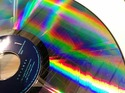Lesson 4

Tape Diagrams`
•••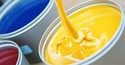•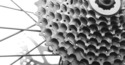Lesson 8

Student Project`
•••Lesson 11

Glide Ratio`
•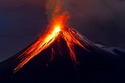•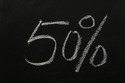Lesson 13

Finding Percents`
••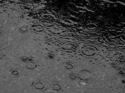•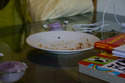•Lesson 17

Gallery Problems`
••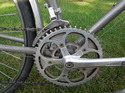Lesson 19

Peer Review`